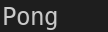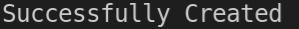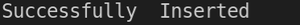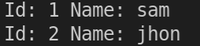Related Articles

# How to Use Go with MySQL?

• Last Updated : 21 Jun, 2021

MySQL is an open-source relational database management system based on Structured Query Language(SQL). It is a relational database that organizes data into one or more tables in which data are related to each other.

Database Driver: A Database Driver implements a protocol for a database connection. The Driver is like an adapter that connects to a generic interface to a specific database.

Initial Setup:

Start MySQL server and install go MySQL driver with the following command.

`go get -u github.com/go-sql-driver/mysql`

Creating database object:

Create a database object with sql.Open. There no connection established with MySQL instead, it creates only a database object which can be used later.

`db, err := sql.Open("mysql", "<user>:<password>@tcp(127.0.0.1:3306)/<database-name>")`

Replace 3306 if not using MySQL on the default port.

## Go

 `package` `main` `import` `(``    ``"database/sql"``    ``"fmt"``    ``_ ``"github.com/go-sql-driver/mysql"``)` `func` `main() {``    ` `    ``// create a database object which can be used``    ``// to connect with database.``    ``db, err := sql.Open(``"mysql"``, ``"root:passwd@tcp(0.0.0.0:3306)/user"``)``    ` `    ``// handle error, if any.``    ``if` `err != nil {``        ``panic(err)``    ``}``    ` `    ``// Now its  time to connect with oru database,``    ``// database object has a method Ping.``    ``// Ping returns error, if unable connect to database.``    ``err = db.Ping()``    ` `    ``// handle error``    ``if` `err != nil {``        ``panic(err)``    ``}``    ` `    ``fmt.Print(``"Pong\n"``)``    ` `    ``// database object has  a method Close,``    ``// which is used to free the resource.``    ``// Free the resource when the function``    ``// is returned.``    ``defer` `db.Close()``}`

Output:fig 1.1

Execute Database Query: A database query can be done with Exec() and Query().

1. Creating a Database Table with SQL query and Exec().

## Go

 `package` `main` `import` `(``    ``"database/sql"``    ``"fmt"` `    ``_ ``"github.com/go-sql-driver/mysql"``)` `func` `main() {` `    ``// create a database object which can be used``    ``// to connect with database.``    ``db, err := sql.Open(``"mysql"``, ``"root:passwd@tcp(0.0.0.0:3306)/user"``)``    ` `    ``// handle error, if any.``    ``if` `err != nil {``        ``panic(err)``    ``}``    ` `    ``//  database object has a method called Exec,``    ``// it executes a database query, but it does``    ``// not return any row as result.``    ``// Here we create a database table with a SQL query.``    ``_, err = db.Exec("CREATE TABLE user(id INT NOT NULL, name VARCHAR(``20``),``                     ``PRIMARY KEY (ID));")``    ` `    ``// handle error``    ``if` `err != nil {``        ``panic(err)``    ``}``    ` `    ``fmt.Print(``"Successfully Created\n"``)``    ` `    ``// database object has  a method Close,``    ``// which is used to free the resource.``    ``// Free the resource when the function``    ``// is returned.``    ``defer` `db.Close()``}`

Output:2. Inserting a row into Database Table with SQL query in Query().

## Go

 `package` `main` `import` `(``    ``"database/sql"``    ``"fmt"` `    ``_ ``"github.com/go-sql-driver/mysql"``)` `func` `main() {` `    ``// create a database object which can be used``    ``// to connect with database.``    ``db, err := sql.Open(``"mysql"``, ``"root:passwd@tcp(0.0.0.0:3306)/user"``)``    ` `    ``// handle error, if any.``    ``if` `err != nil {``        ``panic(err)``    ``}``    ` `    ``// database objec has a method called Query,``    ``// It can execute a SQL query and return rows``    ``// as result. Here we insert a row into  the  table,``    ``// no row returned as result for this operation.``    ``_, err = db.Query(``"INSERT INTO user VALUES(1,'sam')"``)``    ` `    ``// handle error``    ``if` `err != nil {``        ``panic(err)``    ``}``    ` `    ``fmt.Print(``"Successfully  Inserted\n"``)` `    ``// database object has  a method Close,``    ``// which is used to free the resource.``    ``// Free the resource when the function``    ``// is returned.``    ``defer` `db.Close()``}`

Output:3. Using SQL query in Query() to return all rows from the user table.

## Go

 `package` `main` `import` `(``    ``"database/sql"``    ``"fmt"` `    ``_ ``"github.com/go-sql-driver/mysql"``)` `func` `main() {``    ` `    ``// create a database object which can be``    ``// used to connect with database.``    ``db, err := sql.Open(``"mysql"``, ``"root:passwd@tcp(0.0.0.0:3306)/user"``)``    ` `    ``// handle error, if any.``    ``if` `err != nil {``        ``panic(err)``    ``}``    ` `    ``// Here a SQL query is used to return all``    ``// the data from the table user.``    ``result, err := db.Query(``"SELECT * FROM user"``)``    ` `    ``// handle error``    ``if` `err != nil {``        ``panic(err)``    ``}``    ` `    ``// the result object has a method called Next,``    ``// which is used to iterate throug all returned rows.``    ``for` `result.Next() {``        ` `        ``var` `id int``        ``var` `name ``string``        ` `        ``// The result object provided Scan  method``        ``// to read row data, Scan returns error,``        ``// if any. Here we read id and name returned.``        ``err = result.Scan(&id, &name)``        ` `        ``// handle error``        ``if` `err != nil {``            ``panic(err)``        ``}``        ` `        ``fmt.Printf(``"Id: %d Name: %s\n"``, id, name)``    ``}` `    ``// database object has  a method Close,``    ``// which is used to free the resource.``    ``// Free the resource when the function``    ``// is returned.``    ``defer` `db.Close()``}`

Output:My Personal Notes arrow_drop_up